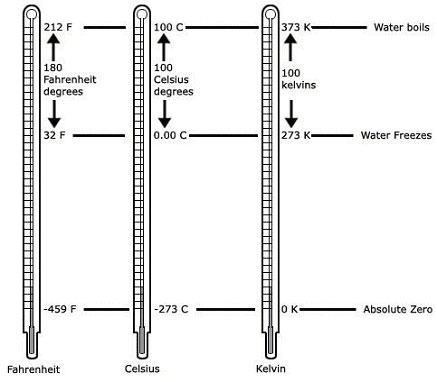A# temperatureComparison of the Fahrenheit, Celsius, and Kelvin scales.

Temperature is the quantity that tells how warm or cold an object is with respect to some standard. It is a measure of the average kinetic energy of the molecules or atoms in an object. The temperature of matter is expressed by a number that corresponds to the degree of hotness on a chosen scale: Celsius (°C), Fahrenheit (°F), or kelvin (K) and is measured quantitatively by thermometers.

## Temperature scales

In most laboratory work, temperature is expressed in degrees Celsius, named after the eighteenth century Swedish astronomer Anders Celsius. On the Celsius scale, zero is assigned to the temperature at which water freezes, and 100 is given to the boiling point of water. The Celsius scale is the same as the centigrade scale.

The United States uses the Fahrenheit scale to express temperatures. In this scale, the value 32 is assigned to the freezing point of water and 212 to its boiling point. Conversion to the Fahrenheit scale from Celsius is through the formula: degrees Celsius = 5/9 × (degrees Fahrenheit - 32).

Scientists also used the kelvin scale, named after the British scientist Lord Kelvin (William Thomson). On this scale, zero is assigned to absolute zero – the lowest possible temperature. At absolute zero, a substance has no kinetic energy. Absolute zero corresponds to -273° on the Celsius scale. The absolute temperature scale, unlike the other temperature scales, is not arbitrary; it is defined with an ideal reversible heat engine working on a Carnot cycle between two temperatures T1 and T2. If Q1 is the heat received at the higher temperature T1, and Q2 the heat lost at the lower temperature T2, the T1/T2 is defined equal to Q1/Q2. Such absolute temperature is independent of the properties of particular substances, and is a basic thermodynamic function, arising out of the zeroth law.

## Temperature and heat

Temperature is an intensive property, unlike heat, which is an extensive property – that is, the temperature of a body is independent of its mass or nature; it is thus only indirectly related to the heat content (internal energy) of the body. Heat flows always from a higher temperature to a lower. On the molecular scale, temperature may be defined in terms of the statistical distribution of the kinetic energy of the molecules.

## Forms of temperature

### antenna temperature

In radio astronomy, antenna temperature is a measure of the power per unit bandwidth (p) of the signal received by an antenna after losses in the detector system. It is defined as p/k, where k is Boltzmann's constant.

In an ideal, loss-free radio telescope, the antenna temperature is equal to the brightness temperature if the intensity of the received radiation is constant within the main lobe. If the angular dimension of the source is small compared to the main lobe, the antenna temperature is equal to the brightness temperature multiplied by the ratio of the solid angle subtended by the source to the effective solid angle of the antenna.

### brightness temperature

The brightness temperature is the temperature that a blackbody would need to have in order to emit radiation of the observed intensity at a given wavelength.

### color temperature

Color temperature is the temperature of a blackbody that has the same color index as a given star.

### critical temperature

The critical temperature is the temperature above which the gas cannot liquefied by pressure alone.

### Curie temperature

For a ferromagnetic material, the Curie temperature (TC) is the temperature above which the material becomes paramagnetic. For iron the Curie temperature is 760°C; for nickel it is 356°C. Also known as the Curie point, it is named after the French physicist Pierre Curie (1859–1906).

### electron temperature

Electron temperature is the temperature determined by the mean kinetic energy of free electrons in a plasma; also known as the kinetic temperature. Inside stars, electron temperature is likely to match the temperature as measured by other methods, but in the rarefied environment of a nebula or the outer atmospheres of stars, it may be quite different.

### ionization temperature

The ionization temperature is the temperature of a gas or plasma derived from the relative numbers of neutral atoms and ions. Specifically, it is the temperature for which the Saha equations would predict these relative numbers, assuming the atoms and ions are in thermodynamic equilibrium.

### kinetic temperature

Kinetic temperature is the temperature of a gas defined in terms of the average kinetic energy of its atoms or molecules. It is given by:

T = 2/3 1/k avg(½ mv 2)

where T is the kinetic temperature, k is Boltzmann's constant, m is the particle mass, and v is the particle velocity. In thermal equilibrium, values of kinetic temperature correspond well with those of other measures of temperature, such as effective temperature; otherwise, they may bear little resemblance.